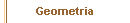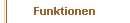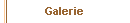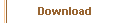Anleitung - GraphenFor the visualisation of mathematical graphs one can use special geometric objects like
vertex, edges or the abstract object graph.

In combination with the JavaScript commands document.Geometria.addObject() and document.Geometria.removeObject(), it is possible to realize the interactive construction of mathematical graphs.

Of course, by using same JavaScript programming this could be extended to define a graph by a matrix, copied into a textarea within the web-page. Some JavaScript functions which are used in the following examples can be found here.

Examples for this feature are given here: